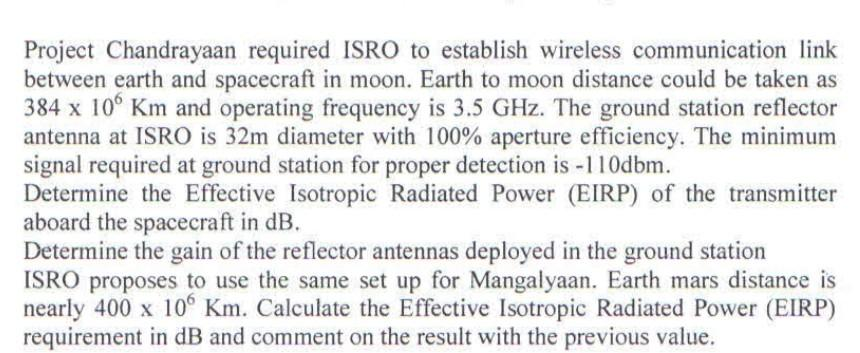# (Solved):   Project Chandrayaan required ISRO to establish wireless communication link between earth a ...Project Chandrayaan required ISRO to establish wireless communication link between earth and spacecraft in moon. Earth to moon distance could be taken as $$384 \times 10^{6} \mathrm{Km}$$ and operating frequency is $$3.5 \mathrm{GHz}$$. The ground station reflector antenna at ISRO is $$32 \mathrm{~m}$$ diameter with $$100 \%$$ aperture efficiency. The minimum signal required at ground station for proper detection is $$-110 \mathrm{dbm}$$. Determine the Effective Isotropic Radiated Power (EIRP) of the transmitter aboard the spacecraft in $$\mathrm{dB}$$. Determine the gain of the reflector antennas deployed in the ground station ISRO proposes to use the same set up for Mangalyaan. Earth mars distance is nearly $$400 \times 10^{6} \mathrm{Km}$$. Calculate the Effective Isotropic Radiated Power (EIRP) requirement in $$\mathrm{dB}$$ and comment on the result with the previous value.

We have an Answer from Expert